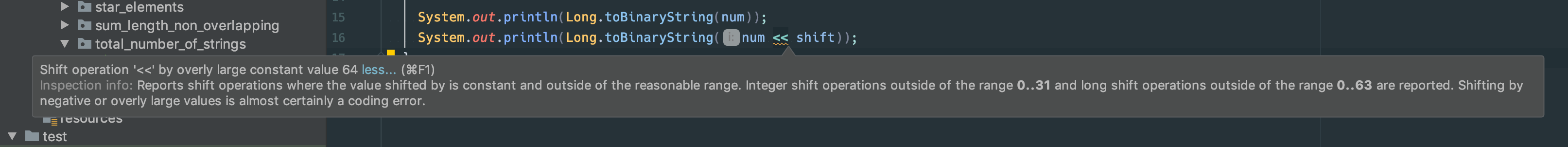Bitwise operation sometimes looks like magic. There is a bunch of sophisticated techniques to manipulate the value at the bit level. Bit shift operation is one of these operations. You can shift the bit sequence of the given value by using shift operators which are commonly described as >> or << in many programming languages. But do you know what happens if you shift the value beyond the length the type of the value in Java?

Let’s say we want to shift the given long value with 64 bit.

long num = 1;
long shift = 63;

System.out.println(Long.toBinaryString(num));
System.out.println(Long.toBinaryString(num << shift));

// 1
// 1000000000000000000000000000000000000000000000000000000000000000


Yes, it’s working fine. The shifted area is padded with zero value. How about shifting 64 bit?

long num = 1;
long shift = 64;

System.out.println(Long.toBinaryString(num));
System.out.println(Long.toBinaryString(num << shift));

// 1
// 1


Umm, it’s a different result from what I expected. I thought we will get zero by shifting 64 bit because all shifted are padded with zero and only one bit will be thrown away. What’s happening?

I found an answer StackOverflow as usual.

Java will shift by num % sizeof(datatype) only, i.e. if you shift an int by 32 it will effectively be no shift at all. If you shift by 33 the effective shift will be 33 % 32 = 1.

The size of the long type is 64 bits so that it does not have any effect on the result by shifting 64 bit. Actually, the specific error message is shown by my IDE. We should shift the long value over 64 bit in general.So please make sure to shift the value within the size of the data type in Java.